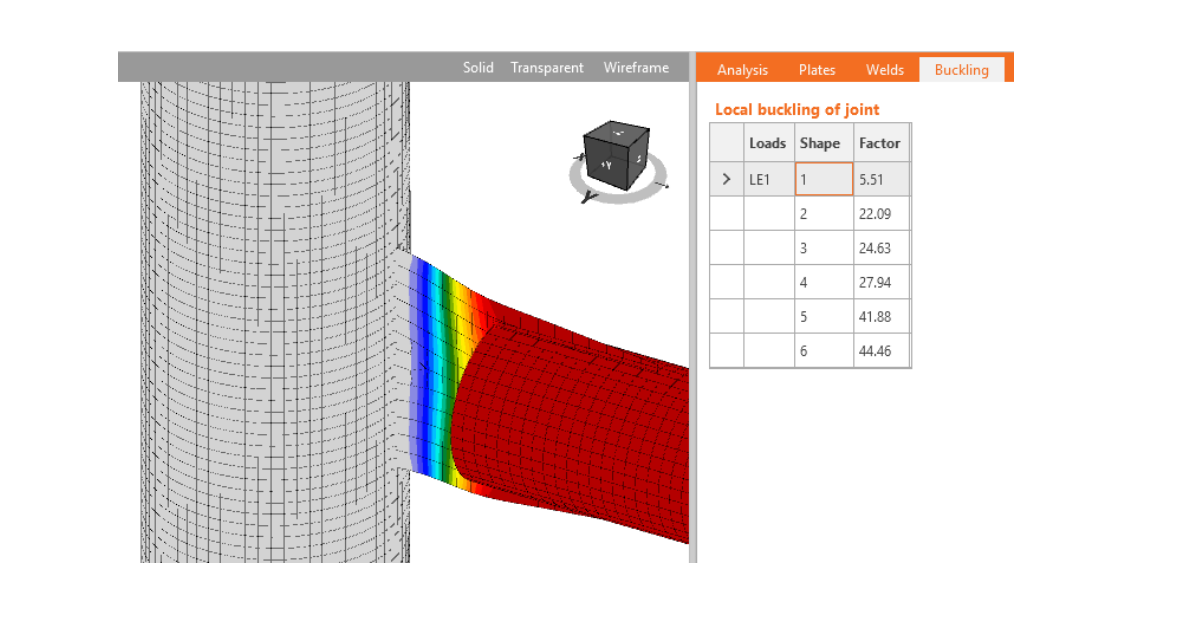Buckling analysis according to AISC

Dit artikel is ook beschikbaar in
$$IDEA Connection allows users to perform linear buckling analysis to confirm the safety of using plastic analysis. The result of linear buckling analysis is buckling factor αcr corresponding to the buckling mode shape. The buckling factor is the multiplicator of set load when Euler’s critical load of a perfect structure is reached. E.g. elastic critical buckling load Pe is determined by: loading a column by compressive force P performing linear buckling analysis, selecting most critical buckling mode (usually the first) and buckling factor αcr multiplying compressive force by buckling factor, i.e. Pe = P × αcr AISC codes use mostly critical slenderness to limit thickness of plates. The critical slenderness can be expressed by critical buckling factor using following formulas:$\lambda = KL/r$$\bar{\lambda_p} = \frac{\lambda}{\pi \cdot \sqrt{\frac{E}{F_y}}}$$\alpha_{cr} = \frac{\alpha_{ult}}{\bar{\lambda_p} ^2} = \frac{\alpha_{ult}}{\left ( \frac{\lambda}{\pi \cdot \sqrt{\frac{E}{F_y}}} \right )^2}$where: λ – plate slenderness KL – effective length r – radius of gyration $$\bar{\lambda_p}$$ – plate relative slenderness $$\alpha_{ult}$$ – minimum load amplifier for the design loads to reach the characteristic value of resistance of the most critical cross section, neglecting any plate buckling and lateral torsional buckling; for load equal to plate plastic resistance, $$\alpha_{ult} = 1$$ E – Young’s modulus of elasticity Fy – yield strength General recommendationIn AISC 360-16 – J.4, it is stated that plastic resistance may be used if λ = KL/r ≤ 25. Then e.g. for steel A36 the corresponding buckling factor is equal to 12.7. Note that for stronger steel, the corresponding buckling factor decreases. That means if buckling factor is higher than 12.7, plastic resistance may be safely used. If the buckling factor is smaller, provisions of Chapter E apply.$\lambda = 25 \ll \gg \bar{\lambda_p} \cong\frac{25}{\pi \cdot \sqrt{\frac{200000}{248.2}}}=0.28$$\alpha_{cr} = \frac{\alpha_{ult}}{\bar{\lambda_p}^2}=\frac{1}{0.28^2} = 12.7$ This limit is very strict and applies generally for all types of plates. It was derived from Dowswell’s research into stability of gusset plates. For gusset plates or connecting plates directly affecting the buckling of connected member, this limit should be used.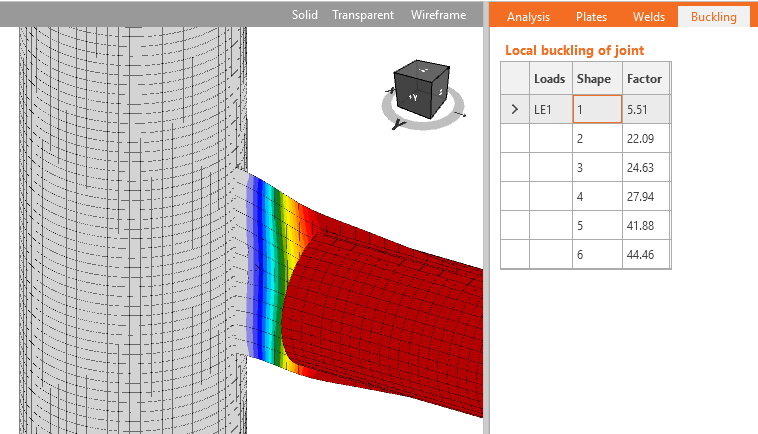Stiffening plates in the jointHowever, for plates in the joint, e.g. stiffeners, haunches, column web panel, the limiting buckling factor may be much smaller using the provisions of Chapter E or corresponding chapters in design guides. A few examples are presented:Limiting width to thickness ratio λr from AISC 360-16, Table B4.1a for web of built-up I section, flange of built-up I section and wall of rectangular hollow section:In the software, the length of standard member was set to 3 and length of member with hollow section to 4 to enable development of local buckling. For these examples, the stiff support for investigated members is necessary and therefore, the strong column fixed on both ends was used. The limiting width-to-thickness ratio is set to the investigated plate. The member is loaded to its compressive resistance. The buckling analysis is performed and the lowest buckling factor corresponding to buckling mode shape for the investigated plate is noted. Other plates in the model are thick so the first buckling mode is relevant. The plate is considered non-slender and its full width can be used. Higher plate thickness leads to higher buckling factor.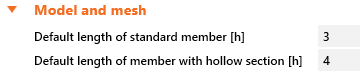E =200000MPaYoung's modulus of elasticity Fy =223.38MPayield strength Fcr =223.38MPacritical stressE3 or E4 Web of built-up I section h =180mmwidth of the element tw =4.1mmthickness h/tw =43.9width-to-thickness ratio for the elementB4.1 λr =44.6limiting width-to-thickness ratioTable B4.1a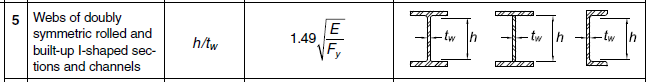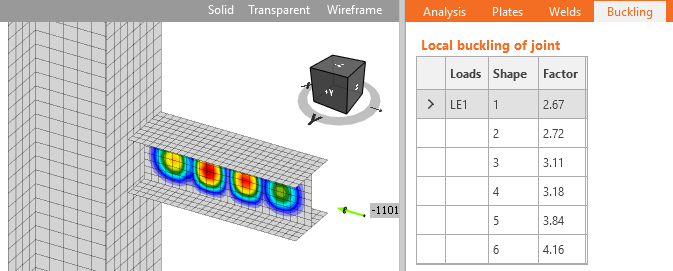Flange of built-up I section b =95mmwidth of the element t =4.7mmthickness b/t =20.2width-to-thickness ratio for the elementB4.1 λr =19.4limiting width-to-thickness ratioTable B4.1a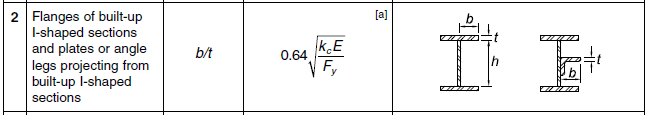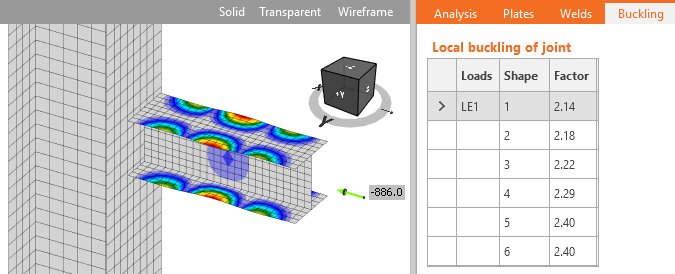Wall of RHS b =180mmwidth of the element t =4.3mmthickness b/t =41.9width-to-thickness ratio for the elementB4.1 λr =41.9limiting width-to-thickness ratioTable B4.1a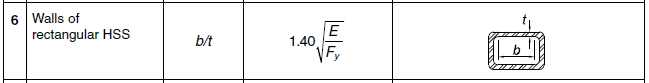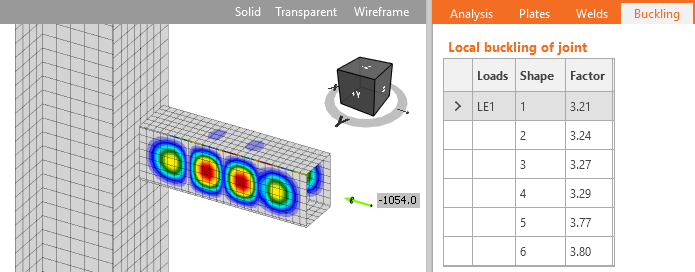Triangular haunch Thickness limitation according to AISC DG4 - 3.16 and AISC 358-18 – 6.8.1 – Step 9:The beam was loaded by a bending moment so that the plate was at its limit compressive strength and then performing linear buckling analysis.End plate stiffener hst =140mmstiffener height ts =8.4mmstiffener thicknessAISC DG4 - 3.16 $\frac{h_{st}}{t_s} \le 0.56 \sqrt{\frac{E}{F_{ys}}} \, \textrm{or} \, t_s \ge 1.79 h_{st} \sqrt{\frac{F_{ys}}{E}} \qquad \textrm{(3.16)}$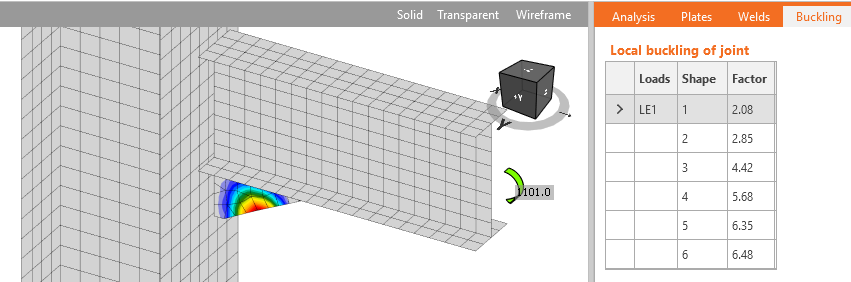The limits in AISC codes correspond for these examples to buckling factor around 3. For experimental research into slender compressed plates in connection see the research papers.ConclusionLinear buckling analysis should be performed if buckling of plates is a possibility in the connection. According to AISC 360-16 – J.4, stability of plates in connections is ensured if slenderness λ ≤ 25, which corresponds to buckling factor αcr = 13. If buckling factor is over 13 no further buckling checks are necessary and plastic analysis may be used without reservations.For plates connecting individual members, e.g. gusset plates, the limit from AISC 360-16 – J.4, αcr ≥ 13, should be used. For stiffening plates in the joint, e.g. stiffeners, ribs, short haunches, the limit of buckling factor may be considered αcr ≥ 3.It is still possible to design a joint with smaller buckling factor but buckling checks must be performed by hand or by geometrically nonlinear analysis with imperfections.ReferencesAISC. (2016). Specification for Structural Steel Buildings. American Institute of Steel Construction, Chicago, Illinois.Dowswell, B. (2006), Effective Length Factors for Gusset Plate Buckling, Engineering Journal, AISC, Vol. 43, No. 2, pp. 91–101.$$